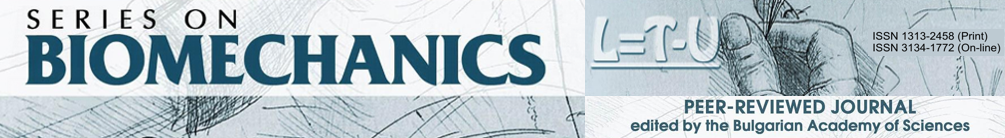bg | enDependence of the elasticity modulus of microplasma coatings made of titanium grade VT1-00 and zirconium grade KTC-110 on their porosity
A. Moltasov, M. Dyman, S. Kaliuzhnyi, I. Mossokovska, S. Voinarovych, O. Kyslytsia, E. Koytchev
Abstract: This study aims to estimate a functional relationship between the modulus of elasticity and porosity of biocompatible coatings made of titanium alloy grade VT1-00 and zirconium alloy grade KTC-110 deposited by the
microplasma method.
The main methods for determining the modulus of elasticity of plasma coatings are analyzed and their advantages and disadvantages are presented. On the basis of the present study, a new experimental-theoretical technique was developed, which makes it possible to determine the elasticity modulus of the coating in bending without separating it from the substrate.
The modulus of elasticity of the coating was determined using static three-point bending (on two supports) and calculating the desired value in the proportional range of the obtained bending diagram.
Regression dependencies that allow determining the elasticity moduli of coatings in the zone of tension and compression were obtained, depending on the degree of their bulk porosity. The functional relationship between bulk porosity and modulus of elasticity was established by constructing linear regression curves using the least squares method.
Mathematical equations were obtained to determine the modulus of elasticity of the coating in explicit form through the geometric dimensions of the coating and substrate, the modulus of elasticity of the substrate, and the experimentally determined flexural stiffness of the entire system; the deviation of the calculated values of the modulus of elasticity from the experimental ones did not exceed 5%.

Series on Biomechanics, Vol.36 No.2 (2022), 141-152
DOI: 10.7546/SB.36.2022.02.14

Keywords: coating; elastic modulus; microplasma sprayed; porosity; Titanium; zirconium
Date published: 2022-06-17
(Price of one pdf file: 29.00 BGN/15.00 EUR)